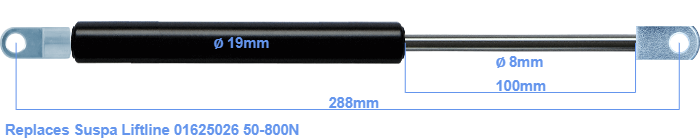# Replacement for Suspa Liftline 01625026 50-800N

\$30.90

Replacement gas spring for the Suspa Liftline 01625026 50-800 Newton. Mounting part tube: The eye has a thickness of 5mm and a hole diameter of 8.1mm. Mounting part rod : The eye has a thickness of 10mm and a hole diameter of 8.1mm. Brand: Stabilus Industry Line.

Please note:
Mounting part rod : The thickness of the eye of this replacement is 10mm instead of 5mm.
 Force Choose an option50 Newton60 Newton80 Newton100 Newton120 Newton140 Newton150 Newton160 Newton180 Newton200 Newton220 Newton240 Newton250 Newton260 Newton280 Newton300 Newton320 Newton340 Newton350 Newton360 Newton380 Newton400 Newton420 Newton440 Newton450 Newton460 Newton480 Newton500 Newton520 Newton540 Newton550 Newton560 Newton580 Newton600 Newton620 Newton640 Newton650 Newton660 Newton680 Newton700 Newton720 Newton740 Newton750 Newton760 Newton780 Newton800 NewtonClear
This gas spring is also known as 16-2 016 25026, 16-2-143-110-A107-B23.The diameter of the cylinder of this gas spring is 19 millimeters. The diameter of the rod is 8 millimeter. The stroke of the gas spring is 100 millimeter. In total, the length is 288 millimeter. Note: this is the total length between the rotation points of the two mounting parts. Without the mounting parts this gas spring is 245 mm long (thread to thread). The force of this replacement gas spring is 50-800 Newton. This gas spring can replace a Suspa Liftline gas spring, but is not an orignal Suspa Liftline one. This gas spring was produced by Stabilus subsidiary Hahn with the brand Stabilus Industry Line. The gas spring can be used without problems as a replacement, because the dimensions and force almost equal.
Category: